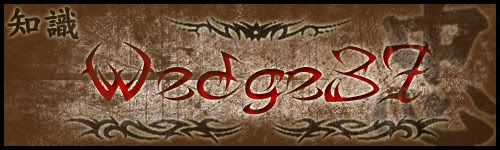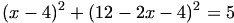Warning: Illegal string offset 'html' in /home/hsn/public_html/forum/cache/skin_cache/cacheid_1/skin_topic.php on line 909

Confusing Straight Line Q - HSN forum# Confusing Straight Line Q

3 replies to this topic

### #1DX_Suck_It

• Gender:Male

Posted 05 September 2006 - 05:28 PM

*ABCD is a square with A the point (2,3) and C(6,5). Find the point of intersection of the diagonals and the co-ords of B and D.

I can't get it, so could somene explain to me how I can solve this question and get the answer, thanks.

### #2DX_Suck_It

• Gender:Male

Posted 05 September 2006 - 08:47 PM

### #3The Wedge Effect

HSN Legend

•• Members
•• 2,477 posts
• Location:Paisley
• Gender:Male

Posted 05 September 2006 - 09:01 PM

I haven't done Higher maths for over a year now, so my memory's a little sketchy, but what you could do to find the point of intersection is use the mid-point formula, as the point of intersection would be at the middle of the two points, as the question states it's a perfect square. You should, as a result, get (4,4) as your answer. However, as I can't remember the distance formula, I'll leave the next part to someone who can, unless there's another way, which I hadn't noticed. God, I am terrible...starting maths at uni, and not remembering something this simple.### #4George

Child Prodigy

••• 720 posts
• Location:West Lothian
• Gender:Male

Posted 05 September 2006 - 10:13 PM

Well, I got an answer, but it was quite involved. I'm maybe not seeing the easiest way, mind you.

Let M be the point where the diagonals meet, so M(4,4).

The diagonal AC has gradient 1/2, so the other diagonal (on which B and D lie) has gradient -2. Since this passes through M, we can write down the equation of the other diagonal:We have dAM2 = 5 (you can check this), and since ABCD is a square, it follows that dBM2 = dDM2 = 5.

Using the pointwhich lies on the other diagonal, this point will be one of B or D if it satisfies(using the distance formula)

Solving this gives two solutions, and these x-values can be put intoto give the coordinates of B and D.

I suspect there is maybe something missing from the question as posted, because that is quite a long path to the answer. Either that, or I'm just doing it the hard way#### 1 user(s) are reading this topic

0 members, 1 guests, 0 anonymous users